# Response times

## A modified version of the dots task

This week we will modify a slightly different version of the dots task in which we can model the response time in addition to the choice.
In this version of the task, participants see a continuous stimulus of dots that does not stop until they indicate their choice. Thus, participants have the option to keep gathering information until they are sure which way the dots are going. This is called the free response version of the dots task, because participants are free to choose when they respond.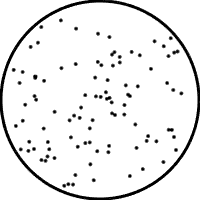In this task we don't just model the choice, but also how quickly that choice is made. Participants (often implicitly, but sometimes explicitly) are under pressure to respond quickly (if you've ever taken part in a perceptual psychology experiment you will understand the desire to just "get out of there as quickly as possible!"). However, responding quickly leads to more chance of making a mistake and participants need to manage a tradeoff between being fast and being correct known as the speed-accuracy tradeoff.
We will see this week how a slight tweak to our simple counting model can model response times in the dots task and account for the speed accuracy tradeoff.

## A modified version of the model

To model response times in the dots task we need to do four things.
1. Compute N_correct and N_wrong on each frame of the movie
2. Compute the difference, Δ between N_correct and N_wrong on each frame of the movie
3. Make a decision difference crosses some threshold.

## Computing N_correct and N_wrong on each frame

we will first reframe our existing model in a slightly different way. Remember how our model worked? We had a direction for each dot (correct direction or wrong direction) and then summed up all the dots moving in the correct direction and wrong direction and made our decision accordingly.
In the makeOneDecision function, this was implemented in Matlab as
function accuracy = makeOneDecision(D, T, f)
% initialize count variables
N_correct = 0;
N_wrong = 0;
% for loop to loop over all dots
for i = 1:D*T
% randomly sample the direction of this dot
dot_direction = binornd(1, f);
% update the counts
N_correct = N_correct + dot_direction;
N_wrong = N_wrong + (1 - dot_direction);
end
% compute accuracy of this choice
if N_correct > N_wrong
accuracy = 1;
end
if N_correct < N_wrong
accuracy = 0;
end
if N_correct == N_wrong
accuracy = binornd(1,0.5);
end
end
Today we are going to reframe this slightly to compute the number of dots moving in the correct direction and the wrong direction on every frame of the movie.
Let's do this in a script first so we can see what's going on and plot things a little easier. First, let's setup the variables we want for this decision
clear
% number of dots
D = 10;
% number of movie frames
T = 20;
% fraction of dots moving in correct direction
f = 0.55;
Then, instead of one loop over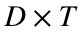dots let's put in two loops over all the dots in each frame and all the frames in each movie like this ...
% initialize count variables
N_correct = 0;
N_wrong = 0;
for i = 1:T
for j = 1:D
% randomly sample the direction of this dot
dot_direction = binornd(1, f);
% update the counts
N_correct = N_correct + dot_direction;
N_wrong = N_wrong + (1 - dot_direction);
end
end
Note that the end result of this is the same as the original code. However, at the end of each iteration of the loop over T, we have the values of N_correct and N_wrong

## Compute the difference

Next we need to take the difference between N_correct and N_wrong on each frame of the movie. To do this we can just add a line to the for loop over T ...
% initialize count variables
N_correct = 0;
N_wrong = 0;
for i = 1:T
for j = 1:D
% randomly sample the direction of this dot
dot_direction = binornd(1, f);
% update the counts
N_correct = N_correct + dot_direction;
N_wrong = N_wrong + (1 - dot_direction);
end
% compute the difference
Delta(i) = N_correct - N_wrong;
end
The vector Delta now contains the difference between the number moving in the correct direction and wrong direction up to the current point in the movie.
Delta
Delta = 1×20
8 6 6 14 16 18 26 24 24 26 30 32 34 38 40 40 42 42 44 46
You can get a bit more intuition for this by plotting it (note I'm using some of the plotting tips from last week to make the plot look nice).
l = plot(Delta);
AZ_chili = [139 0 21]/256;
set(l, 'linewidth', 3, 'color', AZ_chili)
set(gca, 'fontsize', 18)
xlabel('frame number')
ylabel('running difference')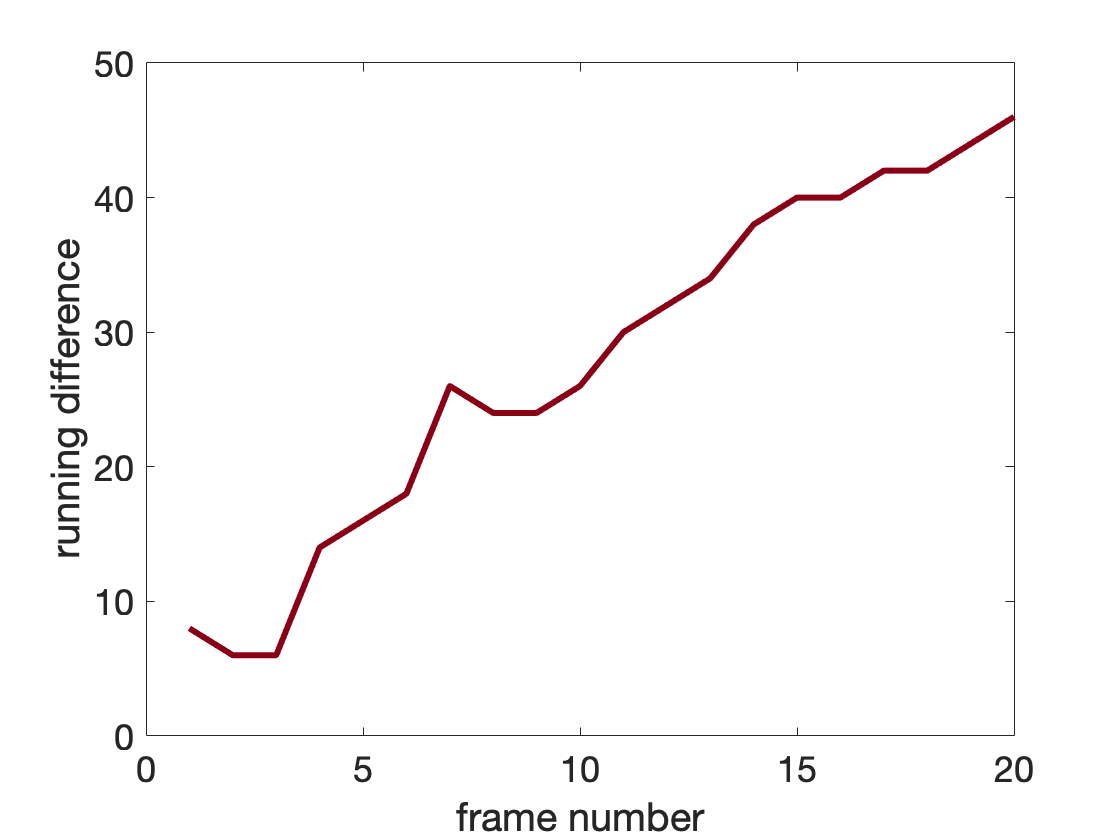You can see that on the early frames the difference is close to zero. This makes sense because there are only 10 dots and there's only a 55% chance of each dot moving in the correct direction so the difference after 1 frame will be close to zero.
However, as time goes on the difference gets bigger (and more positive). This reflects the fact that as I average over more and more frames I'm more likely to get a larger number of dots going in the correct direction than the wrong direction.
In some ways, Δ captures how sure the model is about the decision at each point in the movie. At first, Δ is close to zero and the model isn't particularly sure which way the dots are moving. Later on, the model gathers more and more evidence in favor of the dots going in one direction (usually the correct direction).
This suggests that one way to model the decision in the "free response" version of the dots task is to keep taking in evidence until Δ passes some threshold corresponding to a particular level of "certainty" we need before make the decision.

## Making a decision

So, our idea is to keep looking at the dots until Δ passes some threshold. Let's call the threshold z and let's put one at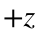(when they make the correct answer) and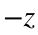when they make the wrong answer. What that means is that if ever the absolute value of Δ gets above z then the model makes a choice.
Now, however, we have a problem. We don't know exactly when Δ will cross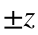. This means we don't know, before we run the code, how many frames of the movie the model will need before it makes its decision.
How can we tell Matlab to keep counting dots until Δ crosses the threshold?
The answer is using a while loop. The intuition for while loops comes from the word itself. While the absolute value of Δ is less than z, keep looping, but stop when the absolute value of Δ gets bigger than z. One way of starting the while loop is like this ...
while abs(Delta(i)) < z
% code we want to repeat
end
where i is the counter that tells us what frame we are on and the "abs" function computes the absolute value of Delta(i).
We then use this loop in place of the for loop over T ...
clear
% number of dots
D = 10;
% fraction of dots moving in correct direction
f = 0.55;
% threshold for decision
z = 40;
% frame counter - note we need to initialize this
% outside the loop now
i = 1;
% initialize the count
N_correct = 0;
N_wrong = 0;
% initialize Delta
Delta(1) = N_correct - N_wrong;
while abs(Delta(i)) < z
for j = 1:D
% randomly sample the direction of this dot
dot_direction = binornd(1, f);
% update the counts
N_correct = N_correct + dot_direction;
N_wrong = N_wrong + (1 - dot_direction);
end
% increment counter by one
i = i + 1;
% compute the difference
Delta(i) = N_correct - N_wrong;
end
% compute accuracy - correct if crossed +z threshold
% wrong if crossed -z threshold
accuracy = Delta(i) > 0
accuracy = logical
1
% compute decision time in frames
DT = i
DT = 50
Note a few things here.
• I had to initialize Delta outside the while loop before I called the line
while abs(Delta(i)) < z
• If I didn't already define Delta, then this line would not work because Delta would not be defined
• I also had to initialize the counter, i and update the counter manually (i = i + 1) in the while loop. You don't need to do this for "for" loops because the counter is defined in the for loop call and updated automatically as the loop is repeated
for i = 1:T
• I got the accuracy of the choice by checking whether the last value of Delta (Delta(i)) was positive or negative.
• I got the decision time, in terms of frames, as the value of i at which the threshold was crossed.
To get a bit more intuition about this process, let's plot Δ over time along with dashed lines for the threshold.
clf;
AZ_chili = [139 0 21]/256;
l = plot(Delta,'.-');
set(l, 'linewidth', 5, 'markersize', 30, 'color', AZ_chili);
hold on;
plot([0 length(Delta)+1], [1 1] * z, 'k--')
plot([0 length(Delta)+1], [1 1] * -z, 'k--')
xlabel('frame number')
ylabel('evidence, \Delta')
set(gca, 'fontsize', 18)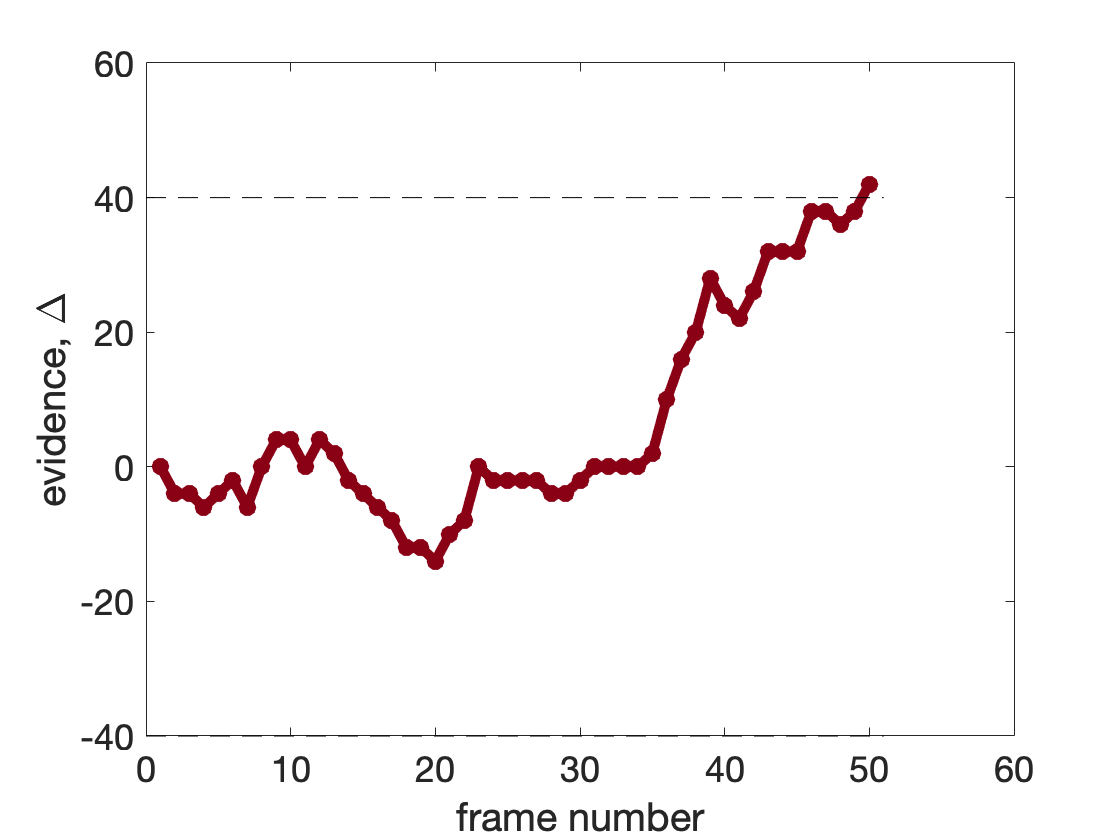What you can see here is how the evidence starts off at zero and gradually increases (at least on average). Note that the last value of Δ is the first value to be above the threshold. This determines the choice (correct in this case) and the decision time, the frame number on which it crossed.

## Making a function to implement the drift diffusion model

Next, let's stick the code to implement the model inside a function (make sure to save it in the same folder as the scripts)
function [accuracy, DT, Delta] = simulate_threshold(D, f, z)
% frame counter - note we need to initialize this
% outside the loop now
i = 1;
% initialize the count
N_correct = 0;
N_wrong = 0;
% initialize Delta
Delta(1) = N_correct - N_wrong;
while abs(Delta(i)) < z
for j = 1:D
% randomly sample the direction of this dot
dot_direction = binornd(1, f);
% update the counts
N_correct = N_correct + dot_direction;
N_wrong = N_wrong + (1 - dot_direction);
end
% increment counter by one
i = i + 1;
% compute the difference
Delta(i) = N_correct - N_wrong;
end
% compute accuracy - correct if crossed +z threshold
% wrong if crossed -z threshold
accuracy = Delta(i) > 0;
% compute decision time in frames
DT = i;
Then let's use this code to simulate a bunch of trials (all with the same D, f, and z
clf
z = 20;
f = 0.55;
for i = 1:10
[choice, DT, Delta] = simulate_threshold(D, f, z);
l = plot(Delta,'-', 'linewidth', 3);
hold on;
end
plot([0 50], [1 1] * z, 'k--')
plot([0 50], [1 1] * -z, 'k--')
xlabel('frame number')
ylabel('evidence, \Delta')
set(gca, 'fontsize', 18)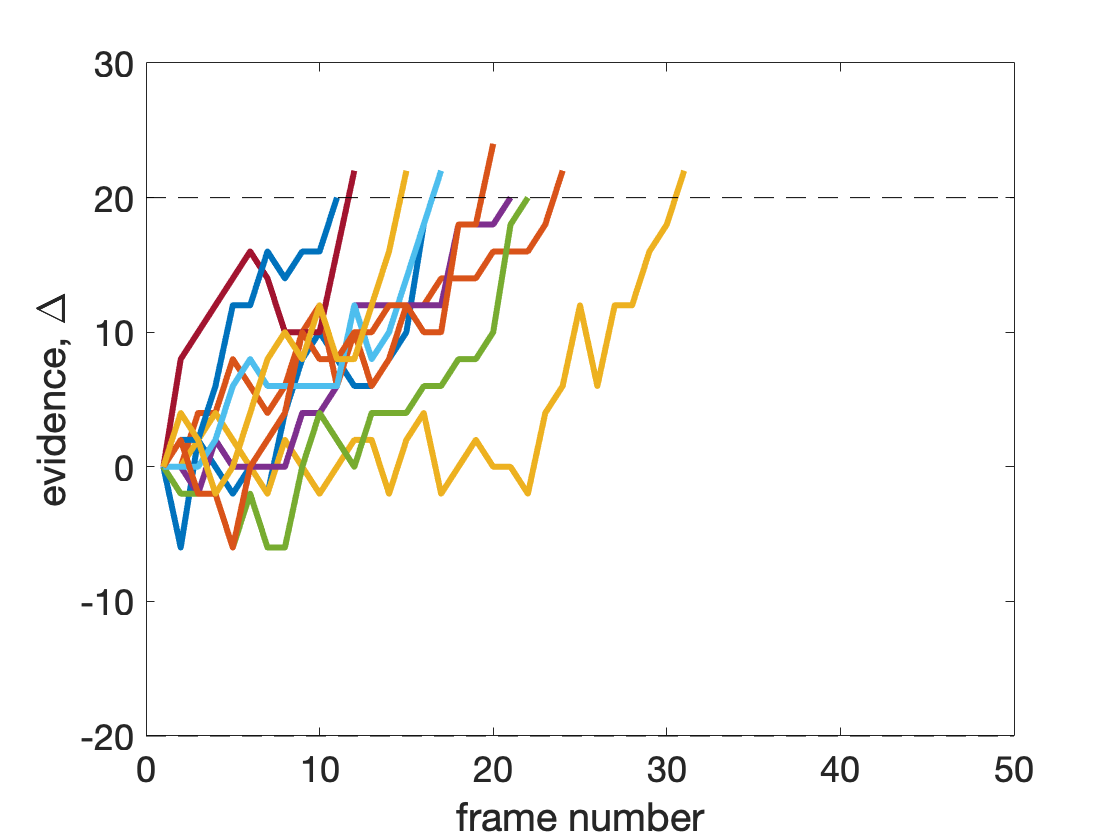From this, you can see that each decision has a different random walk for the evidence, Δ, and a different decision time. Also, you can see that the model mostly seems to cross the top threshold, that is it's making the correct response most times. In general, this model can also make mistakes, but with this relatively high threshold these mistakes are rare. As we will see, mistakes become more frequent when we have a lower threshold.

## The distribution over decision times

What does the distribution over decision times look like? This is something that we often measure in behavioral experiments and it tends to have a pretty characteristic shape ... a bit like this, taken from Bogacz et al. 2006 ...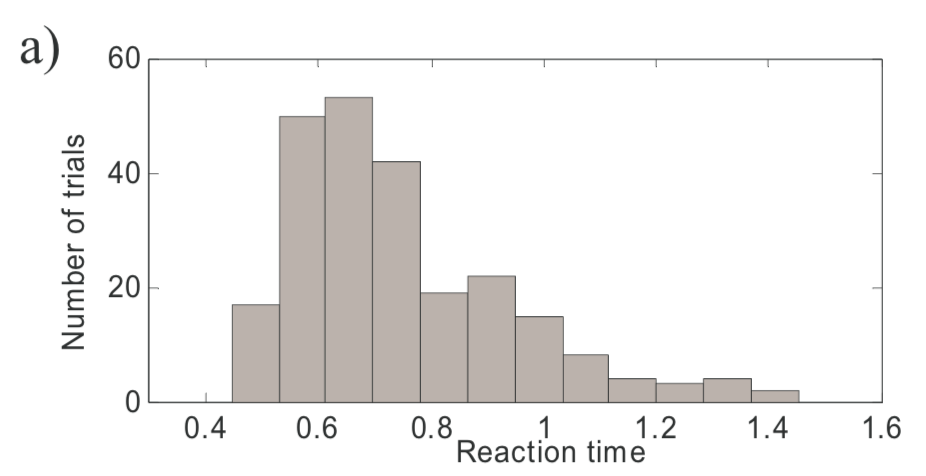Doing the same thing for our simple model, we can get something (at least qualitatively) similar ...
for i = 1:1000
[choice, DT(i)] = simulate_threshold(D, f, z);
end
clf;
hist(DT, 20)
xlabel('response time')
ylabel('number')
set(gca, 'fontsize', 18)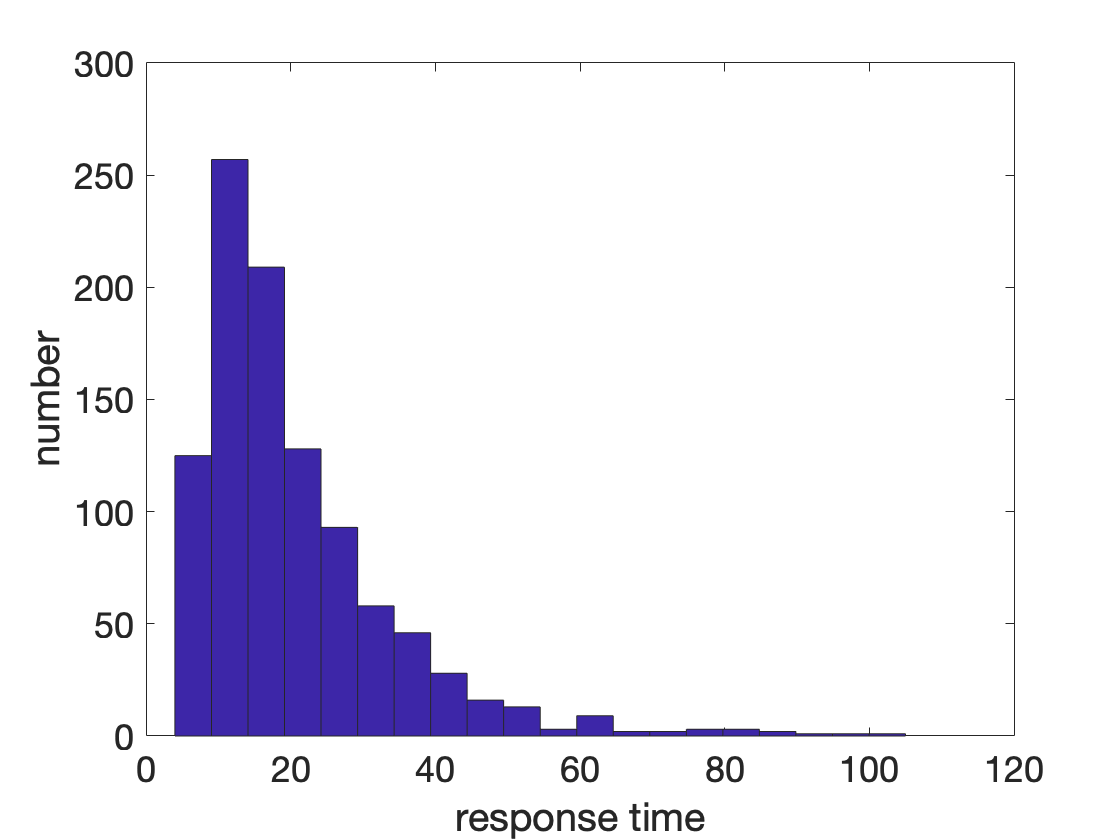This is a really nice feature of the model and it turns out that a slightly more complex form of this model can do a pretty good (but not quite perfect) job of fitting the reaction time distributions in this and other tasks.

## The speed-accuracy tradeoff in the model

Finally, let's demostrate how the model exhibits the speed-accuracy tradeoff. Hopefully you can already see how this might work, when the threshold is small, the model is more likely to respond quickly, but just by chance, the wrong threshold is more likely to be crossed. Conversely, when the threshold is larger, the model is more likely to cross the correct threshold, but will take more time to get there.
To see this with the code, let's simulate the average accuracy and average response time as a function of threshold, z ...
z_vals = [2 4 8 16 32];
for j = 1:length(z_vals)
for i = 1:100
[choice(i), DT(i)] = simulate_threshold(D, f, z_vals(j));
end
meanACC(j) = mean(choice);
meanDT(j) = mean(DT);
end
Then plot the results. First, for z vs accuracy ...
clf
plot(z_vals, meanACC)
xlabel('threshold, z')
ylabel('average accuracy')
set(gca, 'fontsize', 18)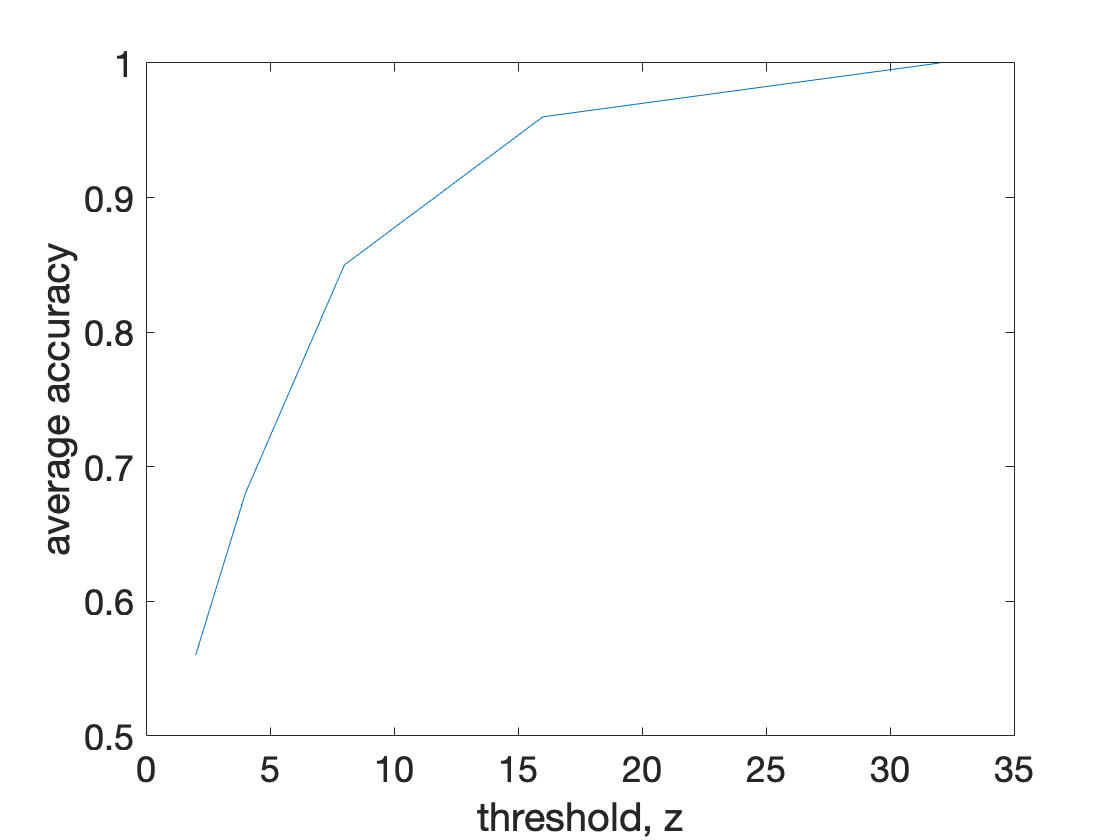As the threshold increases the average accuracy also increases.
Next for z vs decision time ...
clf
plot(z_vals, meanDT)
xlabel('threshold, z')
ylabel('decision time')
set(gca,'fontsize', 18)